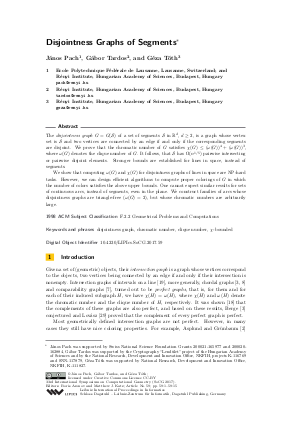Document# Disjointness Graphs of Segments

### Authors János Pach, Gábor Tardos, Géza Tóth## File

LIPIcs.SoCG.2017.59.pdf
• Filesize: 442 kB
• 15 pages

## Cite As

János Pach, Gábor Tardos, and Géza Tóth. Disjointness Graphs of Segments. In 33rd International Symposium on Computational Geometry (SoCG 2017). Leibniz International Proceedings in Informatics (LIPIcs), Volume 77, pp. 59:1-59:15, Schloss Dagstuhl - Leibniz-Zentrum für Informatik (2017)
https://doi.org/10.4230/LIPIcs.SoCG.2017.59

## Abstract

The disjointness graph G=G(S) of a set of segments S in R^d, d>1 is a graph whose vertex set is S and two vertices are connected by an edge if and only if the corresponding segments are disjoint. We prove that the chromatic number of G satisfies chi(G)<=omega(G)^4+omega(G)^3 where omega(G) denotes the clique number of G. It follows, that S has at least cn^{1/5} pairwise intersecting or pairwise disjoint elements. Stronger bounds are established for lines in space, instead of segments. We show that computing omega(G) and chi(G) for disjointness graphs of lines in space are NP-hard tasks. However, we can design efficient algorithms to compute proper colorings of G in which the number of colors satisfies the above upper bounds. One cannot expect similar results for sets of continuous arcs, instead of segments, even in the plane. We construct families of arcs whose disjointness graphs are triangle-free (omega(G)=2), but whose chromatic numbers are arbitrarily large.
##### Keywords
• disjointness graph
• chromatic number
• clique number
• chi-bounded

## Metrics

• Access Statistics
• Total Accesses (updated on a weekly basis)
0

## References

1. Paola Alimonti and Viggo Kann. Some APX-completeness results for cubic graphs. Theoretical Computer Science, 237(1-2):123-134, 2000.2. Edgar Asplund and Branko Grünbaum. On a coloring problem. Mathematica Scandinavica, 8(1):181-188, 1960.3. Claude Berge. Färbung von Graphen, deren sämtliche bzw. ungerade Kreise starr sind (Zusammenfassung). Wiss. Z. Martin-Luther-Univ. Halle Wittenberg Math. Natur. Reihe, 114, 1961.4. Béla Bollobás. Modern Graph Theory, Graduate Texts in Mathematics vol. 184. Springer-Verlag, New York, 1998.5. James P. Burling. On coloring problems of families of prototypes. (PhD thesis), University of Colorado, Boulder, 1965.6. Sergio Cabello, Jean Cardinal, and Stefan Langerman. The clique problem in ray intersection graphs. Discrete &computational geometry, 50(3):771-783, 2013.7. Robert P. Dilworth. A decomposition theorem for partially ordered sets. Annals of Mathematics, pages 161-166, 1950.8. Gabriel Andrew Dirac. On rigid circuit graphs. In Abhandlungen aus dem Mathematischen Seminar der Universität Hamburg, volume 25, pages 71-76. Springer, 1961.9. Gideon Ehrlich, Shimon Even, and Robert Endre Tarjan. Intersection graphs of curves in the plane. Journal of Combinatorial Theory, Series B, 21(1):8-20, 1976.10. Paul Erdős and András Hajnal. Some remarks on set theory. ix: Combinatorial problems in measure theory and set theory. Michigan Math. J, 11(2):107-127, 1964.11. Hillel Furstenberg and Yitzhak Katznelson. A density version of the hales-jewett theorem. Journal d’Analyse Mathématique, 57(1):64-119, 1991.12. Martin Grötschel, László Lovász, and Alexander Schrijver. Geometric algorithms and combinatorial optimization. Springer-Verlag, Berlin, 1988.13. András Gyárfás. On the chromatic number of multiple interval graphs and overlap graphs. Discrete mathematics, 55(2):161-166, 1985.14. András Gyárfás. Corrigendum. Discrete mathematics, 62(3):333, 1986.15. András Gyárfás. Problems from the world surrounding perfect graphs. Applicationes Mathematicae, 19(3-4):413-441, 1987.16. András Gyárfás and Jenő Lehel. Hypergraph families with bounded edge cover or transversal number. Combinatorica, 3(3-4):351-358, 1983.17. András Gyárfás and Jenő Lehel. Covering and coloring problems for relatives of intervals. Discrete Mathematics, 55(2):167-180, 1985.18. András Hajnal and János Surányi. Über die auflösung von graphen in vollständige teilgraphen. Ann. Univ. Sci. Budapest, Eötvös Sect. Math, 1:113-121, 1958.19. György Hajós. Über eine Art von Graphen. Internationale Mathematische Nachrichten, 11:65, 1957.20. Ian Holyer. The NP-completeness of edge-coloring. SIAM Journal on Computing, 10(4):718-720, 1981.21. Gyula Károlyi. On point covers of parallel rectangles. Periodica Mathematica Hungarica, 23:105-107, 1991.22. Gyula Károlyi, János Pach, and Géza Tóth. Ramsey-type results for geometric graphs, i. Discrete &Computational Geometry, 18(3):247-255, 1997.23. Alexandr Kostochka. Coloring intersection graphs of geometric figures with a given clique number. Contemporary Mathematics, 342:127-138, 2004.24. Alexandr Kostochka and Jan Kratochvíl. Covering and coloring polygon-circle graphs. Discrete Mathematics, 163(1):299-305, 1997.25. Alexandr V. Kostochka. Upper bounds for the chromatic numbers of graphs. Modeli i Metody Optim. (Russian), 10:204-226, 1988.26. Jan Kratochvíl and Jaroslav Nešetřil. Independent set and clique problems in intersection-defined classes of graphs. Commentationes Mathematicae Universitatis Carolinae, 31(1):85-93, 1990.27. Jan Kynčl. Ramsey-type constructions for arrangements of segments. European Journal of Combinatorics, 33(3):336-339, 2012.28. David Larman, Jiří Matoušek, János Pach, and Jenő Törőcsik. A Ramsey-type result for convex sets. Bulletin of the London Mathematical Society, 26(2):132-136, 1994.29. László Lovász. Normal hypergraphs and the perfect graph conjecture. Discrete Mathematics, 2(3):253-267, 1972.30. László Lovász. Kneser’s conjecture, chromatic number, and homotopy. Journal of Combinatorial Theory, Series A, 25(3):319-324, 1978.31. László Lovász. Combinatorial problems and exercises. American Mathematical Soc., 1993.32. Torsten Mütze, Bartosz Walczak, and Veit Wiechert. Realization of shift graphs as disjointness graphs of 1-intersecting curves in the plane. Manuscript, 2017.33. Arkadiusz Pawlik, Jakub Kozik, Tomasz Krawczyk, Michał Lasoń, Piotr Micek, William T. Trotter, and Bartosz Walczak. Triangle-free intersection graphs of line segments with large chromatic number. Journal of Combinatorial Theory, Series B, 105:6-10, 2014.34. Svatopluk Poljak. A note on stable sets and colorings of graphs. Commentationes Mathematicae Universitatis Carolinae, 15(2):307-309, 1974.35. Noam Solomon, Michael S. Payne, and Jean Cardinal. Ramsey-type theorems for lines in 3-space. Discrete Mathematics &Theoretical Computer Science, 18, 2016.X

Feedback for Dagstuhl Publishing